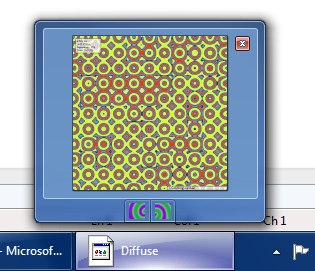# GPU Experiments

A blog which discusses various GPU applications including visualization, GPGPU and games.

## Friday, May 22, 2009

### C++0x Lambdas

I recently installed the VS2010 beta so I could experiment with some of the new C++0x features. In this post, I would like to cover a few simple lambda examples.

Let's start off simple - without lambdas. Suppose we have a vector allocated for 100 floats, and we want to fill it up with random numbers in [0,1). The most obvious way is to use a looping construct of some kind.
``vector<float> vec(100);for (unsigned int i = 0; i < 100; i++){    vec[i] = static_cast<float>(rand()) / (static_cast<float>(RAND_MAX) + 1.0f);}``

Okay, I realize I didn't need that many casts, but I like being safe. ;) While this code does the job, we can also use std::generate() to avoid the explicit loop construct:
``float randval(){    return rand() / (RAND_MAX + 1.0f);}vector<float> vec(100);generate(vec.begin(), vec.end(), randval);``

Looks fine, right? What can we possibly do differently? We can use a lambda:
``vector<float> vec(100);generate(vec.begin(), vec.end(), []() -> float{    return rand() / (RAND_MAX + 1.0f);});``

Now consider the slightly more complicated example where we have two vectors and want to produce a third vector from them. We can use a variant of std::transform() to do this.

We have vectors invec1 and invec2, and want to produce outvec which is simply the elementwise product of invec1 and invec2.

First try:
``float product(float x, float y){    return x * y;}transform(invec1.begin(), invec1.end(), invec2.begin(), outvec.begin(), product);``

Second try:
``transform(invec1.begin(), invec1.end(), invec2.begin(), outvec.begin(), [](float x, float y) -> float{    return x * y;});``

Another application for lambdas is to use one as a sort predicate:
``sort(vec.begin(), vec.end(), [](float x, float y) -> bool { return x > y; });``

Don't forget, we can always assign a lambda to a variable to clean things up a little:
``void somefunc(){    auto f = [](float x, float y) -> bool { return x > y; };    sort(vec.begin(), vec.end(), f);}``

Now for some code to put it all together:
``vector<float> vec(10);// Fill up the vector with random numbers in [0,1)generate(vec.begin(), vec.end(), []() -> float { return rand() / (RAND_MAX + 1.0f); });// Sort the vector in descending ordersort(vec.begin(), vec.end(), [](float x, float y) -> bool { return x > y; });// Print the vectorcopy(vec.begin(), vec.end(), ostream_iterator<float>(cout, " "));cout << endl;``

## Monday, May 4, 2009

### Windows 7 APIs

Windows 7 introduces a number of new APIs. In this post I would like to focus on ITaskbarList3. With this interface, it is possible to turn your application's taskbar button into a progress bar, as well as control what shows up as the thumbnail preview. It is even possible to add buttons to the preview window, as depicted below.The code to add the buttons is quite simple:

``````
DWORD dwMask = THB_TOOLTIP | THB_BITMAP;
THUMBBUTTON tbhButtons;
wstring btn1 = L"Button 1";
wstring btn2 = L"Button 2";

tbhButtons.iId = 0;
tbhButtons.iBitmap = 0;
tbhButtons.szTip[btn1.length()] = L'\0';
btn1.copy(tbhButtons.szTip, btn1.length());

tbhButtons.iId = 1;
tbhButtons.iBitmap = 1;
tbhButtons.szTip[btn2.length()] = L'\0';
btn2.copy(tbhButtons.szTip, btn2.length());